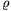Mathematical and Physical Journal
for High Schools
Issued by the MATFUND Foundation
 Already signed up? New to KöMaL?

#Problem P. 4291. (November 2010)

P. 4291. Two soap bubbles are stuck to each other. One half of the gained shape has a radius of R=6 cm and the other half has a radius of r=2 cm.

a) What is the sphere-radiusof the spherical cap which separates the two parts?

b) What is the difference between the pressures in the two parts? The surface tension of the soap solution is (=0.029 N/m.)

c) By what factor is R greater than r in case of two other bubbles which stick together, if the sphere-radius of the cap separating the two parts is R?

(4 pont)

Deadline expired on December 10, 2010.

Sorry, the solution is available only in Hungarian. Google translation

Megoldás. $\displaystyle a)$ $\displaystyle \varrho=\frac{Rr}{R-r}=3~\rm cm.$

$\displaystyle b)$ $\displaystyle \Delta p=\frac{4\alpha}{\varrho}=4~\rm Pa.$

$\displaystyle c)$ $\displaystyle R=2r.$

### Statistics:

 40 students sent a solution. 4 points: Antalicz Balázs, Bajnok Eszter, Barta Szilveszter Marcell, Béres Bertold, Bolgár Dániel, Damokos József, Dávid Bence, Dinev Georgi, Filep Gábor, Fonyó Viktória, Horicsányi Attila, Jéhn Zoltán, Kánvási Dániel, Kollarics Sándor, Kovács 444 Áron, Magyari Sarolt, Maknics András, Nagy Dániel Bálint, Pázmán Koppány, Seress Dániel, Szabó 928 Attila, Szemes Gábor Bence, Varga 515 Balázs, Várnai Péter. 3 points: Csahóczi 222 Márton, Dénes Benjámin, Gargauly Gergő János, Kovács 255 Márton, Laczkó Zoltán Balázs, Pusztaházi 124 Luca Sára, Takács 737 Gábor, Vécsey Máté, Vuchetich Bálint, Wiandt Zsófia. 2 points: 2 students. 1 point: 1 student. 0 point: 1 student. Unfair, not evaluated: 2 solutionss.

Problems in Physics of KöMaL, November 2010